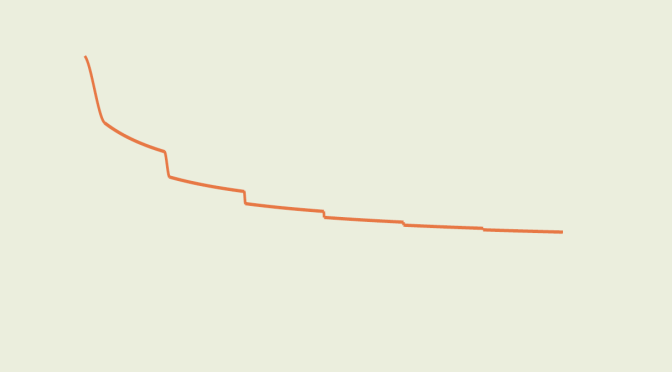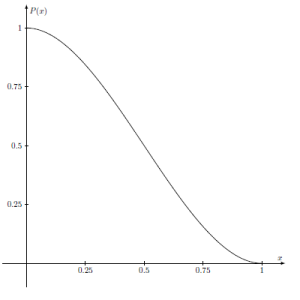A decreasing function converging to zero whose derivative diverges (part2)

In that article, I gave examples of real valued functions defined on $$(0,+\infty)$$ that converge to zero and whose derivatives diverge. But those functions were not monotonic. Here I give an example of a decreasing real valued function $$g$$ converging to zero at $$+\infty$$ and whose derivative is unbounded.

We first consider the polynomial map:
$P(x)=(1+2x)(1-x)^2=1-3x^2+2x^3$ on the segment $$I=[0,1]$$. $$P$$ derivative equals $$P^\prime(x)=-6x(1-x)$$. Therefore $$P$$ is decreasing on $$I$$. Moreover we have $$P(0)=1$$, $$P(1)=P^\prime(0)=P^\prime(1)=0$$ and $$P^\prime(1/2)=-3/2$$.The graph of polynomial $$P$$

The intermediate function $$f: [0,+\infty) \rightarrow \mathbb{R}$$ is defined piecewise as follow:
$f(x) = \begin{cases} \frac{1}{2^{n+1}} [1+P(4^{n+1}(x-n))] & \text{ for } x \in [n,n+\frac{1}{4^{n+1}})\\ \frac{1}{2^{n+1}} & \text{ for } x \in [n+\frac{1}{4^{n+1}},n+1)\\ \end{cases}$ where $$n \in \mathbb{N}$$.
For any $$n \in \mathbb{N}$$, $$f$$ is continuous on the segments $$[n,n+\frac{1}{4^{n+1}})$$ or $$[n+\frac{1}{4^{n+1}},n+1)$$. Considering right and left limits of $$f$$ at points $$n$$ and $$n+\frac{1}{4^{n+1}}$$ for $$n \in \mathbb{N}$$, one can prove that $$f$$ is continuous on $$[0,+\infty)$$. Also $$f$$ is non-increasing as $$f$$ is non-increasing on each of its definition segments.

$$f$$ is differentiable on all segments $$(n+\frac{1}{4^{n+1}},n+1)$$ where $$n \in \mathbb{N}$$ as $$f$$ is constant on those segments and hence $$f^\prime(x)=0$$ there. $$f$$ is also differentiable on all segments $$(n,n+\frac{1}{4^{n+1}})$$ as the composition of $$P$$ which is differentiable on $$(0,1)$$ and a linear function. Let’s analyze the differentiability of $$f$$ at points $$n \in \mathbb{N}$$. For $$0 < h < 1/4^{n+1}$$ we have $$\frac{f(n+h)-f(n)}{h}=\frac{P(4^{n+1}h)-1}{2^{n+1}h}=\frac{-3(4^{n+1})h+2(4^{n+1})^2h^2}{2^{n+1}}$$. Therefore $$\lim\limits_{h \rightarrow 0^+}\frac{f(n+h)-f(n)}{h}=0$$ proving that $$f$$ is differentiable at $$n$$ with $$f^\prime(n)=0$$. Similarly the reader will prove that for $$n \in \mathbb{N}$$, $$f$$ is differentiable at $$n+\frac{1}{4^{n+1}}$$ with again $$f^\prime(n+\frac{1}{4^{n+1}})=0$$. Also, for $$x \in (n,n+\frac{1}{4^{n+1}})$$ we have $$f^\prime(x)=2^{n+1}P^\prime(4^{n+1}(x-n))$$. In particular for $$x=n+\frac{1}{2}\frac{1}{4^{n+1}}$$ we have $$f^\prime(n+\frac{1}{2}\frac{1}{4^{n+1}})=-3\cdot2^n$$ which proves that $$f^\prime$$ is unbounded on $$[0,+\infty)$$. $$f$$ is non-increasing but not decreasing. However it is easy to derive from $$f$$ a decreasing function $$g$$ that converges to $$0$$ and whose derivative is unbounded... just consider $$g(x)=f(x)+1/{(x+1)}$$.